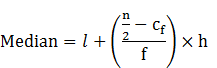Guru

# The distributions of below give a weight of 30 students of a class. Find the median weight of a student. Q.7

• 0

What is the simple way for using the problem of question no.7 of exercise 14.3 of statistics chapter in easy and simple way, give me the best and simple way for solving this problem The distributions of below give a weight of 30 students of a class. Find the median weight of a student.

Share

1. Given: n = 30 and n/2= 15

Median class = 55-60

l = 55, Cf = 13, f = 6 & h = 5Median = 55+((15-13)/6)×5

Median=55 + (10/6) = 55+1.666

Median =56.67

Therefore, the median weight of the students = 56.67

• 0